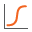Join one of our upcoming courses taking place around the world! Explore now

# Cumulative Plot

A Cumulative Distribution is, mathematically speaking, the integral of the normalized probability density function. Practically speaking, a point on the cumulative distribution gives us the probability that a random variable will be LESS THAN OR EQUAL TO a specified value.

That is, if (X,Y) is a point on the cumulative distribution S-curve, then Y = the probability that the random variable will be <= X.

To plot a Cumulative Distribution after a Probabilistic Analysis:

1. Select Cumulative Plotfrom the toolbar or the Statistics menu.
2. In the Cumulative Plot dialog, select the data you would like to plot. The following data can be plotted:
• Any Random Variable that you have defined for the Probabilistic Analysis (these will appear first in the drop-down list of Data to Plot).
• The Factor of Safety for any analysis method which was used (these will be listed after the input data Random Variables).
3. The Number of Bins used to create the plot can be customized if desired.
4. Select the Plot button to generate the Cumulative plot. Note:
5. The mean, standard deviation, minimum and maximum values for the data plotted, will be listed at the bottom of the plot. If you have plotted Factor of Safety, then the Probability of Failure (PF = ##) and Reliability Index (RI = ##) will also be listed.

6. Once a plot is generated, there are many different options available, if you right-click the mouse on the plot. See below for details.

## Sampling a Cumulative Plot

A useful feature of Cumulative Plots in Slide2 is the ability to easily sample the values at any point on the curve. This can be done graphically, as follows:

1. Right-click on a Cumulative Plot, and select the Sampler option.
2. You will see a dotted vertical line on the plot. This line is the Sampler. The Sampler displays the coordinates of points on the Cumulative Plot, where the Sampler intersects the curve.
1. If you click the LEFT mouse button anywhere on the plot, the Sampler will jump to that location, and display the coordinates at the selected location.
2. If you click AND HOLD the left mouse button, and drag the mouse, the Sampler will follow the mouse, and continuously display the coordinates of points on the curve.
3. The Sampler can be used either horizontally or vertically. If you wish to use the Sampler horizontally, right-click the mouse on the plot, and select Horizontal Sampler. The Sampler operates in the same manner, whether it is used Horizontally or Vertically.

An alternative to the graphical procedure described above is as follows:

1. Right-click on the plot, and select the Sample Exact Value option.
2. In the dialog, select the Specify Value option for the desired axis, and enter a value.
3. Select OK, and the Sampler will display the exact coordinates, at the value specified in the dialog.

TIP: if you are viewing a Cumulative Plot of Safety Factor, the Cumulative Probability at Factor of Safety = 1, is equal to the Probability of Failure.

## Right-Click Options

Other options are available if you right-click on a Cumulative Plot. For example:

• Select the Change Plot Data option to change the data viewed on the plot.
• Select the Chart Properties option to customize the appearance of the plot.

Experiment with the different options available in the right-click menu.